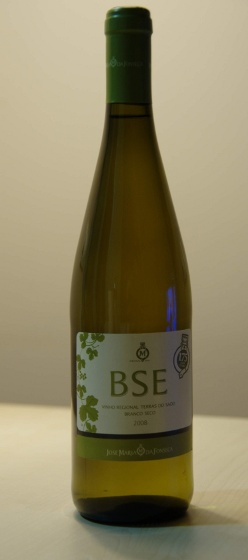The Fortran Cafe'The Bethe-Salpeter-Equation (BSE) wine

TDDFT vs Bethe-Salpeter equation ALDA and long range kernels in 0,1,2 and 3 dimensions

This tutorial describes how to calculate the optical properties of 0/1/2 and 3 dimensional systems in the BS/TDDFT framework.

Before starting the detailed description of the different tutorial examples please read the general remarks about running yambo in the main documentation page.

Subjects

The basic elements needed in the tutorial are the pre-calculated yambo input databases and a list of suggested input files, that the user can edit and modify. The input files are labeled by an increasing number that reflects the different level of approximation used

Note that even if the general physical message can be captured using the quick-to-run input files provided a realistic calculation always require careful convergence tests for all the calculation parameters.

Systems

Click on the material following the order and

1. check the conventions page.
2. run all the provided input files following the given instructions.
3. start from the default input files to create new ones in order to solve the questions posed at the end of each page.
4. To be consistent with the tutorial notation please run any input_file using yambo -F input_file -J input_file.LiF (3D)Si(111)2x1 (almost 2D)Si Nano Wire (almost 1D)H2 molecule (0D)